You may recall in part 1 of this blog series I talked about how I'd forgotten all of my highschool physics knowledge that could help me make canvas animations. It turns out I'd forgotten all my trigonometry as well. It also turns out, that trigonometry is super useful for creating canvas animations and creative coding in general. Awesome. Well done past me.

Luckily for me, I have Google, and I was able to re acquaint myself with "solving triangles" thanks to this wonderful website aptly titled "Math is Fun".

Sometimes, especially for those of us starting out in creative coding, it isn't very obvious as to how or why we'd use this sort of math in our animations. Today I want to show you an example of how we can use trig in combination with our hack physics to create a fun animation.

We're going to make a lever or catapult that the user can pull down with their mouse and fling little penguins in to the air. I know that sounds very exciting. I made it exciting on purpose so you would stick with me through the math stuff. Let's do this.

### As Tina Turner once said, What's love a triangle got to do with it?

The first thing we'll need for our animation, is a lever. The base part is simple enough, we can draw a semicircle at the bottom of our canvas to create this.

```  ```function drawBase() {
ctx.beginPath();
ctx.arc(leverPosition.x, leverPosition.y, 40, Math.PI, 0, false);
ctx.closePath();
ctx.lineWidth = 5;
ctx.fillStyle = '#c1c0c8';
ctx.fill();
}
```
```

Now we need to draw the actual lever. We know we want a 200px long lever, and we're going to have it point out of the base at a 70° angle from the 'ground'. To draw a line on the canvas, you need to know the start point (x1,y1) and the end point (x2,y2). So far we know our start point (the base), but we don't know the end point in the x/y space.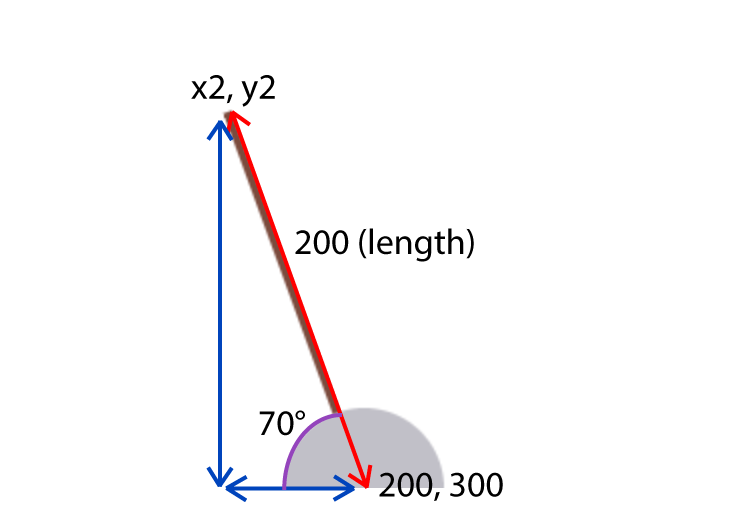When we look at the diagram above we see the lever, x distance, and y distance to our second point actually make the sides of a triangle. We know the lever distance, and the angle, but we need to solve for the x and y sides. Enter trigonometry!

Here's our lever again, broken down in to three angles: A, B & C and three sides: a, b & c.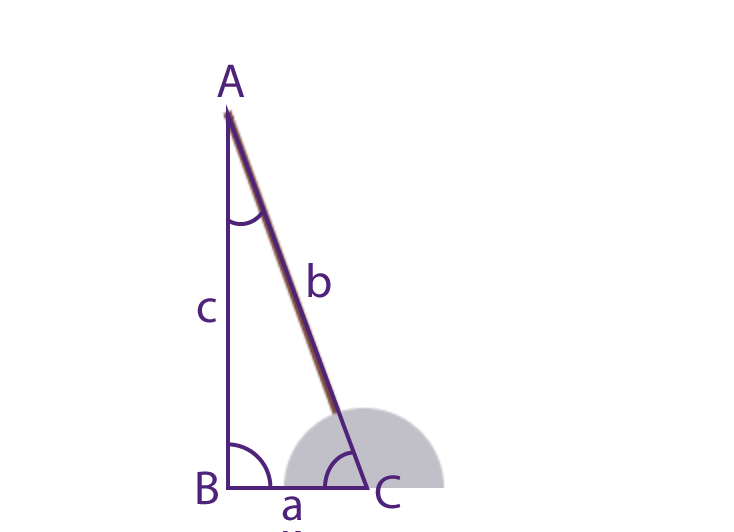The first trig thing we need to know to solve this triangle is that the three angles in a triangle always add up to 180°.

A + B + C = 180

In this case we know angle C (70°) and angle B (a right angle - 90°) so we can solve for angle A.

A = 180 - B - C

Now we have all three angles we can attempt to solve our a (x) and c (y) sides. To do this we can use the The Law of Sines (or Sine Rule).

a / sin(A) = b / sin(B) = c / sin(C)

We have the values for angle C, angle B & side b so we can solve side c.

c / sin(C) = b / sin(B) c = b / sin(B) * sin(C)

Finally, we can solve side a

a / sin(A) = c / sin(C) a = c / sin(C) * sin(A)

Here is a JavaScript function that can find the x/y sides of our lever triangle given an angle C. Don't forget, JavaScript trigonometry functions such as Math.sin require angles to be in radians, and we've been dealing in degrees. I like to use a simple helper function help me keep track of conversions.

```  ```function calculateTriangleXY(C) {
var B = 90;
// solve for A
var A = 180 - C - B;
// solve c and a
var c = (leverLength * Math.sin(toRadians(C))) / Math.sin(toRadians(B));
var a = (c * Math.sin(toRadians(A))) / Math.sin(toRadians(C));
// return x/y sides
return {x: a, y: c}
}

return deg / 180 * Math.PI;
}
```
```

Now we have a function to solve the x/y sides of the triangle, we can draw our lever by subtracting those values from our base point to get the endpoint of the line.

```  ```function drawLever() {
var xy = calculateTriangleXY(currentAngle);
ctx.beginPath();
ctx.moveTo(leverPosition.x, leverPosition.y);
ctx.lineTo(leverPosition.x - xy.x, leverPosition.y - xy.y);
ctx.lineWidth = 4;
ctx.strokeStyle = '#7b4b3d';
ctx.stroke();
}
```
```

We have a lever, now we need to let the user interact with it. The way we can do this is by recording mouse movement and checking if the mouse has connected with the 'hit area' of the lever. However, the 'hit' area in question is a long skinny rectangle on an angle, so it is not as simple as checking if the mouse position is inside a normal x/y bounding box.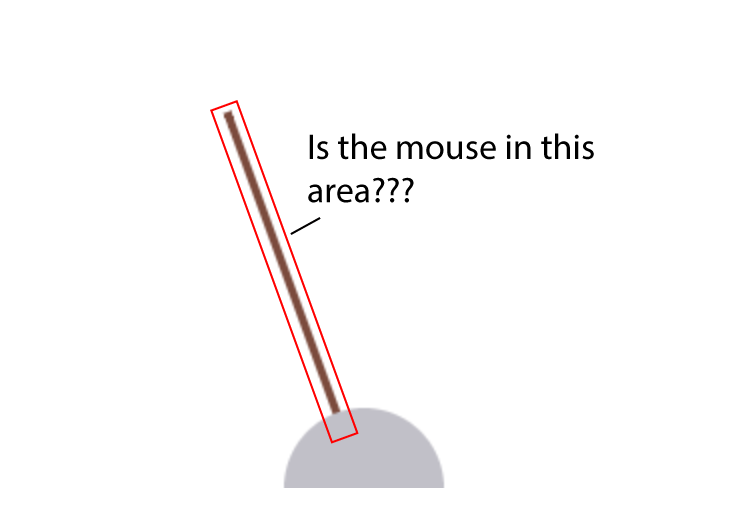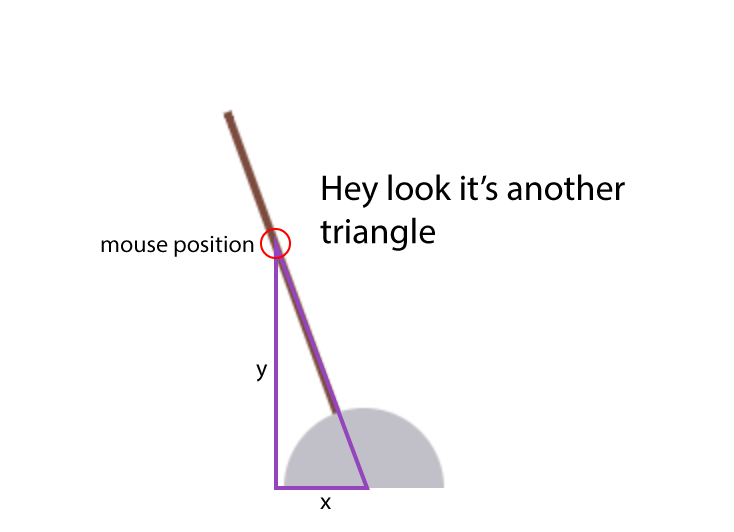We can use trigonometry to solve this problem too! When the user has the mouse in the hit area of the lever it creates another triangle with an angle of 70°. Because we know the y position of the mouse and the angle of the lever, we can check what the x position should be if our mouse is in the hit area. If our mouse x is close to the triangle x then we can assume it is in the hit area. Let's update our `calculateTriangleXY` function so we can pass in a y-position as well as an angle, to get the x position.

```  ```function calculateTriangleXY(C, y) {
var B = 90;
// solve for A
var A = 180 - C - B;
// if we only have angle C we need to solve edge c first
var c = y || (leverLength * Math.sin(toRadians(C))) / Math.sin(toRadians(B));
var a = (c * Math.sin(toRadians(A))) / Math.sin(toRadians(C));
return {x: a, y: c}
}
```
```

Now we can check the mouse position, and if it's in the hit area, we'll change the cursor to a pointer so the user knows it is clickable, and set a variable `hitting` to true.

```  ```// get x given current angle & y pos
var xy = calculateTriangleXY(currentAngle, leverPosition.y - mousePos.y);
// if mouse x is close to the triangle x, we're in the hit area
if ((mousePos.x > leverPosition.x - xy.x - 20) && (mousePos.x < leverPosition.x - xy.x + 20)) {
canvas.style.cursor = 'pointer';
hitting = true;
} else {
canvas.style.cursor = 'auto';
hitting = false;
}
```
```

Now we know when the user has the mouse in the hit area! Awesome! This means that when they click down we can start the 'dragging' of the lever. When the user clicks and drags, we need to make the lever match the position of the mouse. To do this - we need to determine the angle of the lever given the x/y position of the mouse. We can solve this angle using - you guessed it - more trigonometry.

In this example we know the a & c sides thanks to the x/y position of the mouse, and the B angle (90°)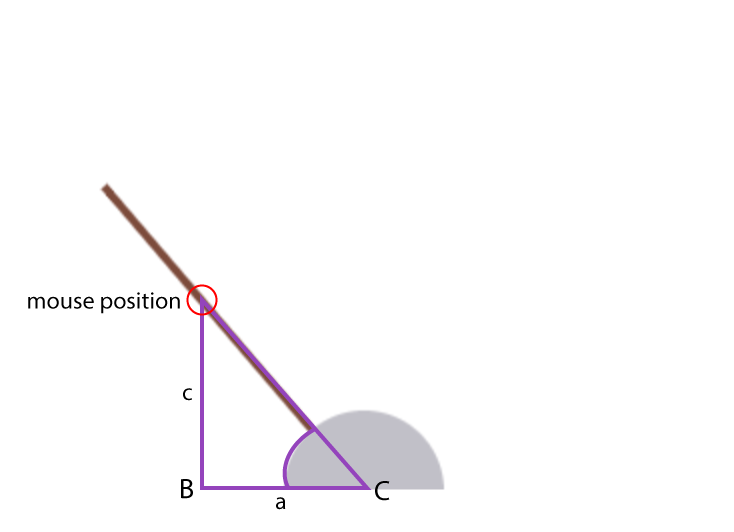To solve for the C angle, we first need to solve for the b side. To do this we can use The Law of Cosines.

b2 = a2 + c2 − (2 * a * c * cos(B))

Once we have the b side, we can solve for the C angle. Here's the function to do that:

```  ```function calculateTriangleAngle(x,y) {
var A, C;
var B = 90;
var c = y;
var a = x;
var b = Math.sqrt(Math.pow(a, 2) + Math.pow(c, 2) - 2 * a * c * Math.cos(toRadians(B)));
C = Math.asin(Math.sin(toRadians(B)) / b * c);
return C;
}
```
```

Then we can set the lever angle to match, resulting in a dragging motion with the mouse!

```  ```var angle = toDegrees(calculateTriangleAngle(leverPosition.x - mousePos.x, leverPosition.y - mousePos.y));
if (angle < 10) {
release();
return;
}
if (angle > 80) angle = 80;
currentAngle = angle;
```
```

Finally, we're going to add a function to create the 'release' of the lever, either when the user lets go or drags the lever all the way to the bottom. Remember in part 1 of this blog when I explained you can have velocity in the x and y direction? You can also have a velocity of rotation, which is what we're setting here. We're going to set a `vr` relative to how far the lever was pulled down, so it heads back to our target angle of 70°

```  ```function release() {
pulling = false;
vr = (70 - currentAngle) * 0.06;
}
```
```

Putting all this together, we have a lever we can drag and release!

### Let's make some penguins fly

How are you going there. Are you still with me? There has been a bit of math I know. This blog post is so long I've actually got distracted and eaten three plates of nachos on separate occasions since I started writing it, so you have my permission to take a little break and eat some mexican food.

First we need to create a Penguin object that we can use to generate penguins

```  ```function Penguin(x, y, r) {
var _this = this;
var img;
this.x = x;
this.y = y;
this.r = targetAngle;

(function() {
img = new Image();
img.src = 'https://s3-us-west-2.amazonaws.com/s.cdpn.io/53148/penguin.png';
})();

this.update = function() {
// rotating the penguin
ctx.save();
ctx.translate(_this.x, _this.y);
// translating a little so it sits further down the ledge
ctx.translate(24, -16);
ctx.drawImage(img, -20, -20, 40, 40);
ctx.restore();
}
}
```
```

Now we can create a penguin and position it on the ledge.

```  ```function addPenguin() {
var penguin = new Penguin(140,120);
penguin.latched = true;
penguins.push(penguin);
}
```
```

You'll notice we've set a `latched` property on the penguin to true, this is so the update function will update the rotation of the penguin as the lever is rotated, making it appear if the penguin is 'latched on' to the lever. We need to release the penguin when the lever has returned to our target angle after being released. We can put a check in our `updateRotation` function for this moment and launch the penguin.

```  ```if (released && currentAngle > targetAngle) {
released = false;
throwPenguin();
}
```
```

'Launching' the penguin involves setting a vx, vy and vr to the penguin, this will send the penguin flying in to the air - once we update our `Penguin` update function with some hack physics.

```  ```function throwPenguin() {
}
```
```
```  ```// added to Penguin.update --
// penguin is launched!
if(!_this.latched && _this.vx) {
_this.vy += gravity;
_this.x += _this.vx;
_this.y += _this.vy;
_this.r += _this.vr;
_this.vx *= 0.98;
_this.vy *= 0.98;

// check for hitting edges
if (_this.x + 28 > canvasWidth) {
_this.x = canvasWidth - 28;
_this.vx *= bounce;
_this.vr *= bounce;
}

if (_this.y + 38 > canvasHeight) {
_this.y = canvasHeight - 38;
_this.vy *= bounce;
_this.vr *= bounce;
}

if (Math.abs(_this.vx) < 0.001) _this.vx = 0;
if (Math.abs(_this.vy) < 0.001) _this.vy = 0;
}
```
```

### Check out the finished demo below

Phew! That was quite a bit of JavaScript, but we got there in the end. Now you know how to solve triangles, what cool things can you make with JavaScript in the canvas? I can't wait to see your ideas.

Make sure you check back for part 3 in my posts on hack physics, where I talk about spring physics.

If you have a question or a topic you would like me to write about next, please leave a comment for me a below, send me a tweet at @rachsmithtweets or flick me an email at contact at rachsmith dot com.

11,677 9 81